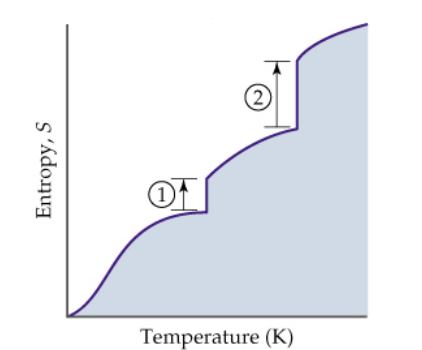# Problem: The diagram below shows the variation in entropy with temperature for a substance that is a gas at the highest temperature depicted. Accordingly, which of the following statements is not true? (a) The “zero point” is explained by the Second Law of Thermodynamics. (b) The entropy of a substance increases with temperature. (c) A solid has the least dispersed energy and the lowest entropy. (d) The vertical line 1 corresponds to ΔSfusion. (e) The increase in entropy for liquid  ⇌ gas is greater than for solid ⇌ liquid

###### FREE Expert Solution
91% (293 ratings)
###### Problem Details

The diagram below shows the variation in entropy with temperature for a substance that is a gas at the highest temperature depicted. Accordingly, which of the following statements is not true?

(a) The “zero point” is explained by the Second Law of Thermodynamics.

(b) The entropy of a substance increases with temperature.

(c) A solid has the least dispersed energy and the lowest entropy.

(d) The vertical line 1 corresponds to ΔSfusion.

(e) The increase in entropy for liquid  ⇌ gas is greater than for solid ⇌ liquidWhat scientific concept do you need to know in order to solve this problem?

Our tutors have indicated that to solve this problem you will need to apply the Entropy concept. You can view video lessons to learn Entropy. Or if you need more Entropy practice, you can also practice Entropy practice problems.

What is the difficulty of this problem?

Our tutors rated the difficulty ofThe diagram below shows the variation in entropy with temper...as medium difficulty.

How long does this problem take to solve?

Our expert Chemistry tutor, Dasha took 4 minutes and 49 seconds to solve this problem. You can follow their steps in the video explanation above.

What professor is this problem relevant for?

Based on our data, we think this problem is relevant for Professor Golightly's class at CAL.